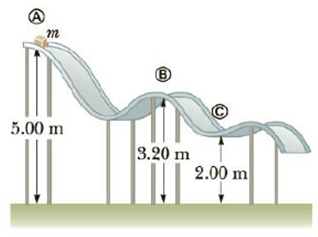Chapter 5, Problem 36P

Chapter
Section
Textbook Problem

A block of mass m = 5.00 kg is released from rest from point Ⓐ and slides on the frictionless track shown in Figure P5.36. Determine (a) the block’s speed at points Ⓑ and Ⓒ and (b) the net work done by the gravitational force on the block as it moves from point from Ⓐ to Ⓒ.Figure P5.36

(a)

To determine
The speed of the block at points B and C.

Explanation

Section1:

To determine: The speed of the block at point B.

Answer: The speed of the block at point B is 5.94m/s .

Explanation:

Given Info:

The mass of the block is 5.0kg .

The height of point A from ground is 5.0m .

The height of the point B from ground is 3.20m .

Since, the track is frictionless; no external force does work on the system.

So according to the conservation of mechanical energy,

12mvB2+mghB=12mvA2+mghA

• vB is the velocity of the block at the point B
• m is the mass of the block
• g is acceleration due to gravity
• hB is the height of the point B
• vA is velocity of the block at point A
• hA is the height of the point A

On re-arranging,

Formula to calculate the speed of the block at B is,

vB=vA2+2g(hAhB)

Substitute zero for vA , 9.8m/s2 for g, 5.0m for hA and 3.20m for hB to find the speed,

vB=0+2(9.8m/s2)(1.80m)=5.94m/s

Thus, the speed of the block at point B is 5.94m/s .

Section2:

To determine: The speed of the block at point C.

Answer: The speed of the block at point C is 7

(b)

To determine
The net work done by the gravitational force when the block moves from point A to C.

Still sussing out bartleby?

Check out a sample textbook solution.

See a sample solution

The Solution to Your Study Problems

Bartleby provides explanations to thousands of textbook problems written by our experts, many with advanced degrees!

Get Started